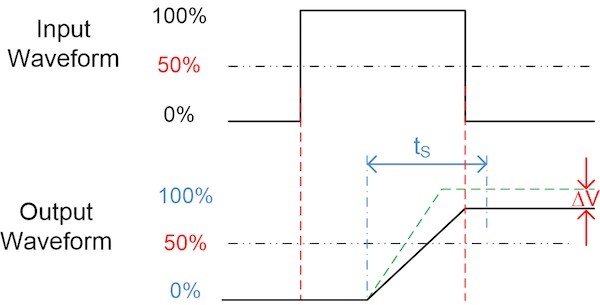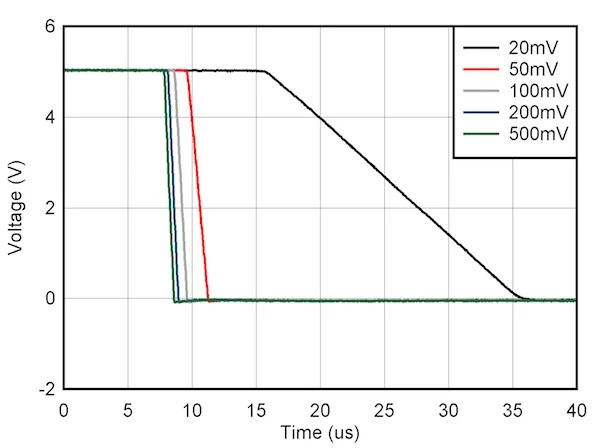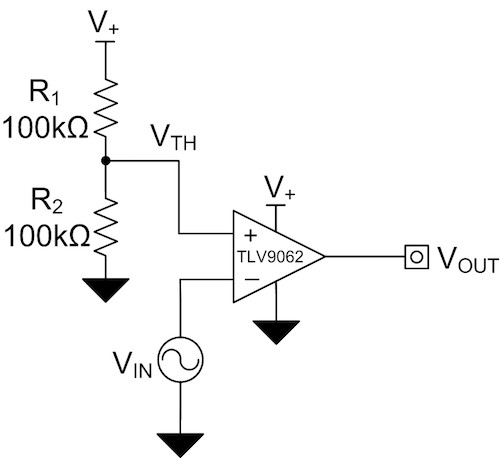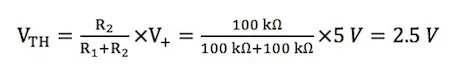### Slew Rate

• Slew rate is the maximum rate of change of an op-amp’s output voltage, thus affecting the rise and fall times of Figure 4’s output waveform. For comparator applications, the output usually needs to change from high to low or low to high once the input voltage crosses the threshold voltage. Slew rate is a key specification because it limits the rate at which the output voltage can change. A lower slew rate takes longer for the output to reach the final amplitude, which may lead to timing errors if the input signal changes before the output voltage can reach a high or low state.
• Figure 6 shows how slew rate affects timing when configuring an op-amp as a comparator. In this example, tS causes tTOTAL to exceed the allowable transition time. The green dashed line displays correct timing when the slew rate is fast enough for the output to reach a high or low state before the input signal changes. The output waveform’s solid line indicates a situation where the device has too slow of a slew rate. Notice that the output does not reach the final amplitude before the input signal changes, leading to potential timing errors, shown as ΔV in Figure 6.• The propagation delay contains effects of the overload recovery time and slew rate, which are each affected by the differential signal amplitude applied to the input. Increasing the differential input voltage or overdrive voltage can reduce the propagation delay time. Figure 7 shows how different amplitudes of input overdrive voltage affect propagation delay on the TLV9062. Notice that the larger the input overdrive voltage, the faster the propagation delay. Overdrive voltages less than 100 mV will result in longer propagation delays because the overload recovery time increases and the slew rate decreases.Figure 7: Input overdrive voltage vs. falling edge propagation delay

Design Procedure

It’s possible to simplify the procedure for designing an op-amp as a comparator into two design steps:

• Set the threshold voltage using a resistor divider or reference voltage.

• Select an op-amp that meets all of the design requirements discussed here.

• Figure 8 shows the TLV9062 configured as an inverting comparator. This device does not have input clamping diodes (a requirement to operating an op-amp as a comparator), features rail-to-rail input and output, and has a slew rate of 6.5 V/µs and an overload recovery time of 200 ns.Figure 8: Comparator application using the TLV9062

• For an inverting comparator topology, connect the input signal, VIN, to the inverting pin of the op-amp and the threshold voltage, VTH, to the noninverting pin of the op-amp. In this configuration, the output of the op-amp transitions high to the positive supply (V+) when the input signal is less than the threshold voltage and transitions low to the negative supply (GND) when the input signal is greater than the threshold voltage.

• A resistor divider, R1 and R2, and the supply voltage, V+, set the threshold voltage for the design. Equation 2 calculates the threshold voltage. Setting resistor R1 equal to R2 sets the threshold voltage to mid-supply.